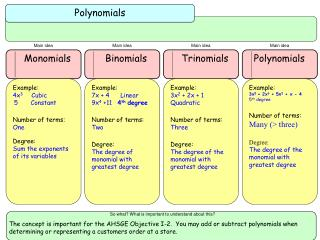DownloadDownload PresentationPolynomials

# Polynomials

Download Presentation## Polynomials

- - - - - - - - - - - - - - - - - - - - - - - - - - - E N D - - - - - - - - - - - - - - - - - - - - - - - - - - -
##### Presentation Transcript

1. Polynomials Main idea Main idea Main idea Main idea Monomials Binomials Trinomials Polynomials Example: 4x3 Cubic 5 Constant Number of terms: One Degree: Sum the exponents of its variables Example: 7x + 4 Linear 9x4 +11 4th degree Number of terms: Two Degree: The degree of monomial with greatest degree Example: 3x2 + 2x + 1 Quadratic Number of terms: Three Degree: The degree of the monomial with greatest degree Example: 3x5 + 2x3 + 5x2 + x - 4 5th degree Number of terms: Many (> three) Degree: The degree of the monomial with greatest degree So what? What is important to understand about this? The concept is important for the AHSGE Objective I-2. You may add or subtract polynomials when determining or representing a customers order at a store.

2. 1.Graph the linear equations on the same coordinate plane. • 2.If the lines intersect, the solution is the point of intersection. • 3.If the lines are parallel, there is no solution. Polynomial Products Multiplying Polynomials and Special Products Main idea Main idea Main idea Main idea Square of a Binomial Binomials times Binomials Difference of Two Squares Polynomials times Polynomials Monomial times a Polynomial: Use the distributive property (x coeff + exponents) Example: -4y2 ( 5y4 – 3y2 + 2 ) = -20y 6 + 12y 4 – 8y2 Polynomial times a Polynomial: Use the distributive Property Example: (2x – 3) ( 4x2 + x – 6) = 8x3 – 10x2 – 15x + 18 FOIL: F = First O = Outer I = Inner L = Last Example: (3x – 5 )( 2x + 7)= 6x2 + 11 x - 35 (a + b) 2 = a2 + 2ab + b2 (a – b) 2 = a2 – 2ab + b2 1st term: Square the first term 2nd term: Multiply two terms and Double 3rd term: Square the last term Examples: (x + 6)2 = x2 + 12 x + 36 (7m – 2p)2 = 49m2 –14mp + 4p2 DOTS: (a + b) ( a – b) = a2 – b2 Example: (t3 – 6) (t3 + 6) = t6 – 36 So what? What is important to understand about this? AHSGE Objective: I -3. Applications include finding area and volume. Special products are used in graphing functions by hand. Punnett Squares in Biology.

3. 1.Graph the linear equations on the same coordinate plane. • 2.If the lines intersect, the solution is the point of intersection. • 3.If the lines are parallel, there is no solution. Solving Systems of Linear Equations Is about … Finding solutions for more than one linear equation by using one of four methods. Method 1 Method 4 Method 2 Method 3 GRAPHING SUBSTITUTION ELIMINATION W/MULTIPLICATION ELIMINATION • Look for variables with opposite or same coefficients. • If the coefficients are opposites, add the equations together. • If the coefficients are the same, subtract the equations, by changing the sign of each term in the 2nd equation and adding. • Substitute the value of the remaining variable back into one of the orig. equations to find the other variable. • 1.Choose a variable to eliminate. • 2.Look the coefficients and find their LCD. This is the value you are trying to get. • 3.Multiply each equation by the needed factor to get the LCD. • 4.Continue as for regular elimination. • Solve one of the linear equations for one of the variables (look for a coefficient of one). • Substitute this variable’s value into the other equation. • Solve the new equation for the one remaining variable. • Substitute this value into one of the original equations and find the remaining variable value. So what? What is important to understand about this? • 1.Graph the linear equations on the same coordinate plane. • 2.If the lines intersect, the solution is the point of intersection. • 3.If the lines are parallel, there is no solution. • 4. If the lines coincide, there is infinitely many solutions The solution (if it has just one) is an ordered pair. This point is a solution to both equations and will test true if substituted into each equation.